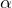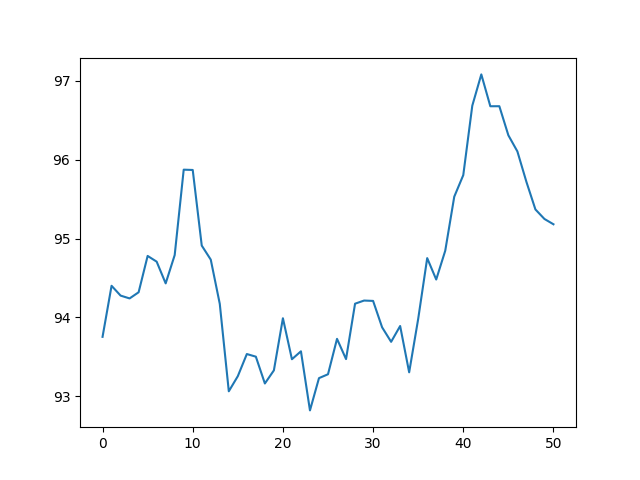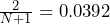# Improving GBM Stock Path Generation With Exponentially Weighted Statistics

In a recent post, I wrote about using Monte Carlo simulations to determine the likelihood of a stock option being profitable by generating multiple paths using Geometric Brownian Motion (GBM) and computing some statistics of these paths. This project can be found on my personal project website. Shortly after finishing that project, I was watching a YouTube video from The Plain Bagel, about volatility in the stock market. In this video, the idea of a stock’s volatility changing rapidly (or even gradually) is brought up and it struck me that using historical statistical properties of a time-series will likely lead to spurious prediction results, especially if these properties are prone to change (a realization likely encountered long ago by professionals in the field).

Immediately, I began thinking of how to improve the predictive capabilities of models relying on historical data and determined one improvement would be to pay more attention to recent data to better capture changes in the time-series’ statistical properties. One way to apply such a weighting is to use exponential moving or exponentially weighted moving statistics. In these calculations, the statistics of the time-series are weighted with the recent values being considered more important and the importance of historical observations decaying exponentially. The moving component of the names comes from the fact that, as new observations become available, the new observation is added and the oldest is removed creating a sliding window effect. As an example of the exponential decay, the weights applied to each sample of N=20 time periods of historical data are shown below (the discount factoris not revealed in the Wikipedia post).

In this post, I will walk through the reasoning behind using weighted samples for computing statistics about time-series data. I will also apply these changes to the stock options tool created in my previous post.

## Changes in Volatility

Consider a company comprised of two divisions or subsidiaries: a slow-growing more mature component with steady profits and a smaller fast-growing division with spurious financial results. Clearly, as individual companies, these two divisions would be valued very differently by investors.

Now, assume that the company announces a sale of its mature business so that the remaining company, i.e. what will continue to be owned by investors, is only the smaller, fast-growing component. Without stable profits from the mature division, the stock should naturally become more volatile as financial results become more variable. This will be reflected in the stock price by wider fluctuations in price

Finally, imagine a trading algorithm dependent on long-term simple moving averages. The increase in volatility will not be reflected in these averages for a considerable amount of time since the old, stable data has more bearing on the average than recent price swings. The problems arising from this issue can be mitigated using exponential moving averages.

## Example With Random Price Fluctuations

Below is a contrived and somewhat extreme example of how this could look in reality. The data below was generated by taking an initial stock value of $100. Data was generated by multiplying each value in the series by a randomly generated percent change. For the first 100 values, the random fluctuations were drawn from a normal distribution parametrized with a mean of 0 and a standard deviation of 0.005 after that the random fluctuations are drawn from a normal distribution with mean 0 and a standard deviation of 0.015 (i.e. 3 times the variance) for 50 more time-series data points. The first 50 data-points are truncated as they are used to get the ball rolling on the 50-period exponential and simple moving averages. The 50 low-variance data points are plotted below.Price of the underlying security in a ‘low-volatility’ regime. As seen here, the price fluctuates but stays with the$93-97 price range; not too volatile. Following these price movements, at time-period 50, there is an assumed change in the fundamentals of the underlying company that makes the stock 3 times more volatile. A graph of the price during this time is shown below.

Notice this graph picks up right where the last one left off; this will be shown more clearly later. In this graph, it’s easily seen that the price fluctuations from period to period are more extreme and this also leads to a wider range of prices for the same number of time periods.

We can mash these graphs together and add some niceties to help visualize this transition into the higher volatility regime.

Also plotted on this graph are the 50-period simple and exponential moving averages. As seen here, the slower-moving 50-period simple moving average (SMA) barely reacts to the changes in volatility but the 50-period exponential moving average (EMA) reacts more readily to the new, wilder fluctuations. Note for those interested, the smoothing factor of the EMA used here was, where N is the number of periods in the moving average.

The graph above helps show that using the exponentially weighted moving average can assist in capturing sudden changes in the dynamics of the underlying time-series generator. In the next section, the geometric Brownian motion tool used to predict future stock price ranges, developed in a previous post, will be updated to use an exponentially weighted moving average and standard deviation.

## Updating the Tool

Fortunately, the Pandas DataFrame object already has built-in functionality to calculate the exponentially weighted functions. This is easily done via

import pandas as pd

df = pd.DataFrame(...)
ewm_variance = df['column'].ewm(alpha=0.94).var()
ewm_avg = df['column'].ewm(alpha=0.94).mean()
ewm_std_dev = df['column'].ewm(alpha=0.94).std()

In the code above, the alpha parameter is the smoothing factor. According to an Investopedia post on the topic, a good smoothing factor (one used by RiskMetrics) is 0.94 so that’s what I’ve chosen here.

Therefore, there are only two places in the logic from the GBM post that need updating. The Stock class’ compute_stats() function

def compute_stats(self, price_header='Close'):

self.avg = self.open_diffs.mean()
self.var = self.open_diffs.var()
self.std = self.open_diffs.std()

# .94 recommended by RiskMetrics (https://www.investopedia.com/articles/07/ewma.asp)
self.emvar = self.open_diffs.ewm(alpha=0.94).var().iloc[-1]
self.ema = self.open_diffs.ewm(alpha=0.94).mean().iloc[-1]
self.emstd = self.open_diffs.ewm(alpha=0.94).std().iloc[-1]

and the simulation’s run() method

past = self.current - relativedelta(days=int(self.data_months*30))
portfolio = dh.get_data_for_tickers(symbols, past, self.current)
for sym in symbols:
portfolio[sym] = Stock(sym, portfolio[sym])

paths = {}
for sym in symbols:
stock = portfolio[sym]
s_0 = stock.get_last_price()

sig = stock.emstd
sig2 = stock.emvar
mu = stock.ema

# random daily shocks to the stock price (price fluctuations)
shocks = {sim: random.normal(0, 1, self.T) for sim in range(self.simulations)}
# cumulative sum of all previous shocks to the stock price (price path)
brownian_motion = {sim: shocks[sim].cumsum() for sim in range(self.simulations)}
paths[stock.get_sym()] = [[s_0] for _ in range(self.simulations)]

Note that full code can be found in my blog post on this topic and these snippets can be used to update that logic. In the code above, the highlighted regions are the parts of the code that have been updated